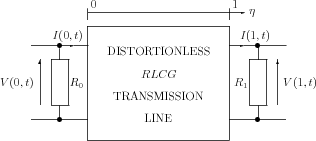]> 8.6 Physical realization of the optimal controller

### 8.6 Physical realization of the optimal controller

By (8.18), the optimal control law is
 $u=-{g}^{#}x=\left(1-p\right){x}_{2}\left(0\right)+\left(b-q\right){x}_{1}\left(-r\right)$ (8.19)

Notice that (8.6) has been introduced to avoid the use of a non–linear nonautonomous operator

${\mathcal{𝒜}}_{n}x={x}^{\prime },\phantom{\rule{1em}{0ex}}D\left({\mathcal{𝒜}}_{n}\right)=\left\{x\in {\text{W}}^{1,2}\left(-r,0\right)\oplus {\text{W}}^{1,2}\left(-r,0\right):\phantom{\rule{0ex}{0ex}}x\left(0\right)-Ax\left(-r\right)={b}_{0}u\left(t\right)\right\}.$

Taking (8.19) into account, we get an abstract operator of the optimal closed–loop system in the form

 $\begin{array}{c}{\mathcal{𝒜}}_{c}x={x}^{\prime },\hfill \\ D\left({\mathcal{𝒜}}_{c}\right)=\left\{x=\left[\begin{array}{c}{x}_{1}\hfill \\ {x}_{2}\hfill \end{array}\right]\in {\text{W}}^{1,2}\left(-r,0\right)\oplus {\text{W}}^{1,2}\left(-r,0\right):\phantom{\rule{0ex}{0ex}}Mx\left(0\right)+Nx\left(-r\right)=0\right\}\hfill \end{array}$ (8.20)

where

$M=\left[\begin{array}{cc}\hfill 1\hfill & \hfill 0\\ \hfill 0\hfill & \hfill p\end{array}\right],\phantom{\rule{1em}{0ex}}N=\left[\begin{array}{cc}\hfill 0\hfill & \hfill -1\\ \hfill q\hfill & \hfill 0\end{array}\right].$

Since our result (8.19) is partially based on some formal considerations, any possibility of checking its validity is of a great interest. Such a possibility arises by examining the eigenproblem of ${\mathcal{𝒜}}_{c}$:

 $\left\{\begin{array}{cccc}\hfill {x}^{\prime }\left(\theta \right)& \hfill =\hfill & \lambda x\left(\theta \right),\hfill & -r\le \theta \le 0\hfill \\ \hfill Mx\left(0\right)+Nx\left(-r\right)& \hfill =\hfill & 0\hfill & \hfill \end{array}\right\}$ (8.21)

Clearly (8.21) has a solution iff $det\left(M+{e}^{-\lambda r}N\right)=p+q{e}^{-2\lambda r}=0$. Hence the closed–loop characteristic function is conﬁrmed to be the numerator of the spectral factor (8.13).

Now let us obtain the physical realization of the optimal feedback for the original system (8.1). To do this, using (8.12) we ﬁnd, that the time–domain version of the optimal feedback in terms of system (8.5) is

 $u\left(t\right)=\left(1-p\right){z}_{2}\left(t\right)+\left(b-q\right){z}_{1}\left(t-r\right)=\left(1-p\right)\left[{z}_{2}\left(t\right)-q{z}_{1}\left(t-r\right)\right]$ (8.22)

Next, recalling (8.4) and again (8.19), we have

 $u\left(t\right)=\frac{1-p}{p}\left[\frac{1}{2}p{e}^{-\alpha t}\Phi \left(-\nu t\right)-\frac{1}{2}{e}^{-\alpha t}\Psi \left(\nu t\right)\right]$ (8.23)

Now observe that, by (3.8),

 $\left\{\begin{array}{ccc}\hfill ZI\left(0,t\right)& \hfill =\hfill & {e}^{-\alpha t}\frac{\Phi \left(-\nu t\right)-\Psi \left(\nu t\right)}{2}\hfill \\ \hfill V\left(0,t\right)& \hfill =\hfill & {e}^{-\alpha t}\frac{\Phi \left(-\nu t\right)+\Psi \left(\nu t\right)}{2}\hfill \end{array}\right\}$ (8.24)

The quantities $\frac{1}{2}{e}^{-\alpha t}\Phi \left(-\nu t\right)$ and $\frac{1}{2}{e}^{-\alpha t}\Psi \left(\nu t\right)$ can be uniquely evaluated from (8.24). Introducing them into (8.23), we get

 $u\left(t\right)=\frac{1-p}{2p}\left[\left(Zp+Z\right)I\left(0,t\right)+\left(p-1\right)V\left(0,t\right)\right]$ (8.25)

But, from the original system (8.1), we know that $u\left(t\right)=V\left(0,t\right)$, hence (8.25) yields the desired expression for the optimal feedback in terms of system (8.1), viz.

 $V\left(0,t\right)=Z\frac{1-{p}^{2}}{1+{p}^{2}}I\left(0,t\right)$ (8.26)

The physical interpretation of (8.26) is astonishingly simple: the optimal feedback is realized by the impedance ${R}_{0}=Z\frac{\left(1-{p}^{2}\right)}{\left(1+{p}^{2}\right)}$ plugged to the input of the distortionless $RLCG$–transmission line – see Figure 8.2.Figure 8.2: The optimal control system